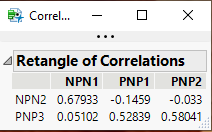Choose Language Hide Translation Bar
Highlighted

## How do I compute correlations: Not quadratic (ABCXYZ by ABCXYZ), but rectangular (ABC by XYZ) (scripting, JSL)

Hi,

This time I may have a simple question. I just cannot find anything fitting.

I simply would like to compute correlations. Not “quadratic”, for example coefficients of columns ABCXYZ by ABCXYZ, but “rectangular”, for example coefficients of columns ABC by columns XYZ. Any ideas how I could accomplish that with JMP?

Nothing fancy...

Cheers!
Newbie2Jumpie

7 REPLIES 7
Highlighted

## Re: How do I compute correlations: Not quadratic (ABCXYZ by ABCXYZ), but rectangular (ABC by XYZ) (scripting, JSL)

I have no idea what you mean by "not quadratic" or "rectangular" but you might check out the Multivariate platform or the Correlation() JSL function. Your choice might depend on where the data is stored (data table or matrix object).

Learn it once, use it forever!
Highlighted

## Re: How do I compute correlations: Not quadratic (ABCXYZ by ABCXYZ), but rectangular (ABC by XYZ) (scripting, JSL)

I just would like to restrict the computations down to two groups of columns (A-F) by (X-Z):

A B C D E F X Y Z

A  ccAA...

B

C

D

E                        81 correlation coefficients

F

X

Y

Z                               ccZZ

"rectangular"

X  Y   Z

A  ccAX ...

B

C           18 correlation coefficients

D

E

F                        ...ccFZ

Highlighted

## Re: How do I compute correlations: Not quadratic (ABCXYZ by ABCXYZ), but rectangular (ABC by XYZ) (scripting, JSL)

Here is a simple script that will produce what you want:``````Names Default To Here( 1 );
dt = Open( "\$SAMPLE_DATA/semiconductor capability.jmp" );

xList = {"NPN1", "PNP1", "PNP2"};
yList = {"NPN2", "PNP3"};

nw = New Window( "Correlations",
Outline Box( "Retangle of Correlations", tb = Table Box() )
);

// Add the Row Labels to the Table Box

tb << append( String Col Box( " ", yList ) );
For( x = 1, x <= N Items( xList ), x++,
xyCorrList = {};
For( y = 1, y <= N Items( yList ), y++,
Insert Into(
xyCorrList,
Correlation(
Column( dt, xList[x] ) << get as matrix || (Column( dt, yList[y] ) <<
get as matrix)
)[1, 2]
)
);
tb << append( Number Col Box( xList[x], xyCorrList ) );
);``````
Jim
Highlighted

## Re: How do I compute correlations: Not quadratic (ABCXYZ by ABCXYZ), but rectangular (ABC by XYZ) (scripting, JSL)

Hi Tx,

The "textbox"  is an interesting, though completely approach to me.

How would I adjust this code to get these coefficients in a JMP table?

JMP, at least v14.0, does not like to do this using "textbox".

Kind regards

Newbie

Highlighted

## Re: How do I compute correlations: Not quadratic (ABCXYZ by ABCXYZ), but rectangular (ABC by XYZ) (scripting, JSL)

I am a little confused about the "textbox" you are refering to.  I think you may be misrefferencing the Table Box() my last response used to create the correlation table.  In any case, the code works fine in JMP 14 or JMP 15  Anyway, below are 2 modifications to the code I previously sent.  Each of them produce a JMP data table as the output for the correlations.

The first one simply takes the original code, and creates a data table from the Table Box() the original code produces.

``````Names Default To Here( 1 );
dt = Open( "\$SAMPLE_DATA/semiconductor capability.jmp" );

xList = {"NPN1", "PNP1", "PNP2"};
yList = {"NPN2", "PNP3"};

nw = New Window( "Correlations",
Outline Box( "Retangle of Correlations", tb = Table Box() )
);

// Add the Row Labels to the Table Box

tb << append( String Col Box( " ", yList ) );
For( x = 1, x <= N Items( xList ), x++,
xyCorrList = {};
For( y = 1, y <= N Items( yList ), y++,
Insert Into(
xyCorrList,
Correlation(
Column( dt, xList[x] ) << get as matrix || (Column( dt, yList[y] ) <<
get as matrix)
)[1, 2]
)
);
tb << append( Number Col Box( xList[x], xyCorrList ) );
);

tb << make into data table;``````

This second script, produces the output data table directly.  Not generating a Table Box() first.

``````Names Default To Here( 1 );
dt = Open( "\$SAMPLE_DATA/semiconductor capability.jmp" );

xList = {"NPN1", "PNP1", "PNP2"};
yList = {"NPN2", "PNP3"};

dt2 = New Table( "Correlations");
dt2 << add rows( N Items( yList ) );
dt2 << delete columns( "Column 1" );dt2 << New Column( "Label", character, values(yList));

For( x = 1, x <= N Items( xList ), x++,
xyCorrList = {};
For( y = 1, y <= N Items( yList ), y++,
Insert Into(
xyCorrList,
Correlation(
Column( dt, xList[x] ) << get as matrix || (Column( dt, yList[y] ) <<
get as matrix)
)[1, 2]
)
);
dt2 << new column( xList[x], values(xyCorrList));
);``````
Jim
HighlightedjulianCommunity Manager

## Re: How do I compute correlations: Not quadratic (ABCXYZ by ABCXYZ), but rectangular (ABC by XYZ) (scripting, JSL)

Looks like you already have a scripting solution from Jim, but I have an Add-in out there that I think does what you want: return a table of correlations between a set of particular Xs and particular Ys rather than all possible combinations (as you would get in Multivariate).

Hope that helps,

Highlighted

## Re: How do I compute correlations: Not quadratic (ABCXYZ by ABCXYZ), but rectangular (ABC by XYZ) (scripting, JSL)

the others gave you a direct answer to your question. let me offer another solution which i think JMP is really good for.

why not try Pairwise correlations under the red triangle (or in preferences). this table can be exported to a data table and then filtered as you like. i find this much more useful than the big correlation matrices where all the numbers appear twice.

Article Labels

There are no labels assigned to this post.